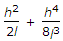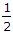# Civil Engineering - Surveying - Discussion

Discussion Forum : Surveying - Section 1 (Q.No. 2)
2.
If h is the difference in level between end points separated by l, then the slope correction is. The second term may be neglected if the value of h in a 20 m distance is less thanm
1 m
2 m
3 m
Explanation:
No answer description is available. Let's discuss.
Discussion:
66 comments Page 1 of 7.

Khagendra said:   2 years ago
For slope (M) up to 20%.
Slope correction(Sc) = h^2/(2l).
Here d = 20m,
M = h/d,
0.2 = h/20.
H = 0.2 * 20 = 4m.
Here the nearest one is 3m.
So 3m is the appropriate answer.
(5)

Nandhu said:   3 years ago
The second term can be negleted only if the slopes flatter than 1 in 25..
That means 4%.

i.e, 4% of 20m = 0.8(4/5) ie, H shouldn't higher than 0.8m.
So option A is right.
(2)

LATHA said:   5 years ago
H^2=2*20.
=6.32.

SUBSTITUTE THE VALUE IN THE FORMULA.
6.32^2/2*20+6.32^4/8*(20^3).
0.998+2.492=3.4 (approx).
(1)

Mukesh das said:   2 years ago
How it will be 3m?

Please explain clearly with the completed formula.
(1)

Dev Narayan Yadav said:   2 years ago
l = 20 m.
H2 = 2l,
H2 = 2*20,
H2 = 40.
And;
H = 6.32 or 6.33,
√40 = 6.32.
(1)

Vaibhav rana said:   6 years ago
@All.

Very simple put tan(3°)=h/20.
h=1m.

Tapas Kumar Dinda said:   4 years ago
H^4/8L^3 = 0 or near to 0 which is neglected to measure, when I put h<3 (1/2,1,2), results are below 1mm, which is hard to measurable. So, 3 is correct.

Durga said:   5 years ago
How can you get 3? Please explain the solution in detail.

Ghalib said:   5 years ago
For slope less than 1 in 25 so h/l is less than or eqal to1 in 25 so h is q.

Equal to or less than (.8).

Aniket said:   5 years ago
In (h^4) / (8l^3), The Value comes out 0.008 (Almost 0%) up to filling of h=2.9. At h=3m the value comes out 0.01.

so, h=3 is the right answer.